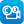Close message Scootle will be undergoing maintenance between 1:00 pm to 2:00 pm today, 25 November 2021. You may experience intermittent connection at this time.

# Search results

## Refine by topic

Main topic
Specific topic
Related topic
Listed under:  Science  >  Matter  >  Properties of matter  >  Mass
Listed under:  Science  >  Mechanical energy  >  Mass### Gravity and orbits

This is an interactive teaching and learning resource that years 7 to 10 secondary students can use to simulate the orbits of the Earth, Moon and a space station while altering the physical quantities involved. Orbital pathways, velocity and force vectors can be displayed in either scale or cartoon views. The mass and velocity ...### reSolve: Statistics - Licorice Lines

This sequence of two lessons explores the concept of statistical variation. Students make licorice logs both by hand and by using a Play Doh machine. They record and compare the attributes of both types of licorice by plotting their findings and comparing the shapes of the two plots. Students are then challenged to produce ...### Energy skate park: basics

This is an interactive teaching and learning resource that years 7 to 10 secondary school students can use to simulate the motion of a skateboarder descending and ascending on a variety of tracks. Height, speed and energy conservation are visually displayed. The skater's mass and starting height, as well as the drag he ...### reSolve: Measurement - Parcel in the Post

This lesson challenges students to find the most cost-efficient way to package a variety of items to send in the post. Students are provided with a matrix of parcel postage charges and a variety of different possible parcels. They categorise the parcels, measure their dimensions and weights and then calculate the postage ...### reSolve: Algebra - Equivalence

This sequence of two lessons builds students' understanding of equivalence as balance. The equals sign is used to indicate the same value on both sides of an equation. Students develop their understanding of equivalence by looking at balancing scales with blocks of different weights. Each lesson is outlined in detail including ...### TIMES Module 10: Measurement and Geometry: introduction to measurement - teacher guide

This is a 16-page guide for teachers. It provides an introduction to the initial ideas of measurement, and introduces the measurement of length, area, volume and time.### Density Simulation (sk-Intel)

This resource is in the style of an 'authentic' scientific investigation. The investigation is set in a crime lab where finding the densities of the various items can solve the crime. The tool enables students to explore mass and volume for a variety of solids and liquids and hence determine their densities.### Gravity

Students use this resource consisting of four slides with diagrams, written explanation and voice-over to understand that gravity is a force of attraction which acts on Earth towards the centre of the planet, and that the size of the force of gravity depends on the mass of each object and the distance between them. There ...### Sites2See – measurement for primary

Selected links to a range of interactive and print resources for Measurement topics in K-6 Mathematics.